Posted on

# MATHEMATICS FORM 2 TOPIC 2: ALGEBRA## UNAWEZA JIPATIA NOTES ZETU KWA KUCHANGIA KIASI KIDOGO KABISA:PIGA SIMU:0787237719

When we play games with computers we play by running, jumping and or finding secret things. Well, with Algebra we play with letters, numbers and symbols. And we also get to find secret things. Once we learn some of the ‘tricks’ it becomes a fun challenge to work with our skills in solving each puzzle. So, Algebra is all about solving puzzles. In this chapter we are going to learn some of the skills that help in solving mathematics puzzles.
The Binary Operations
Describe the binary operations
When two numbers are combined according to the instructions given and produce one number we say that they are binary operations. For example, when we add 4 to 6 we get 10, or when we multiply 4 by 3 we get 12. We see that addition of two numbers lead to one number and multiplication of two numbers produce one number. This is binary operation. The instructions can be given either by symbols like X,*,∇and so on or by words.
Performing Binary Operations
Perform binary operations
Example 1
Evaluate: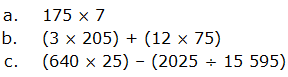solution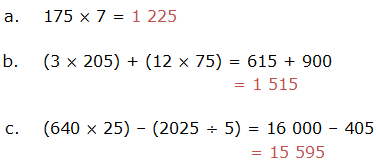Example 2
Find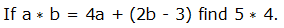solution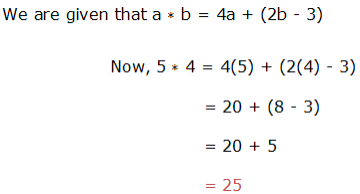Example 3
Solve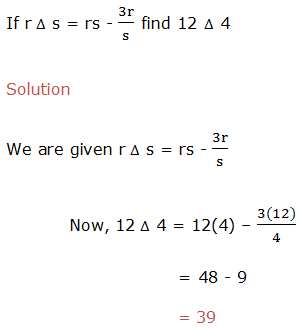Example 4
evaluate,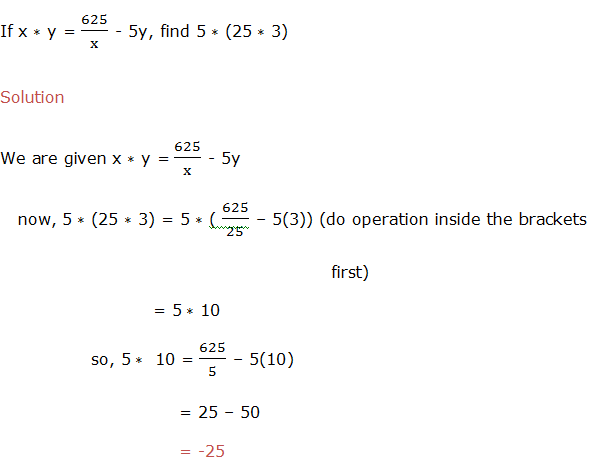Example 5
Calculate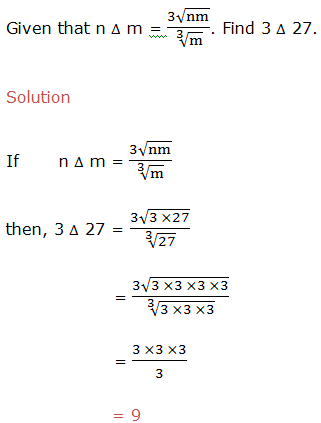Linear Expressions
Factorize linear expressions
The operation of resolving a quantity into factors, when we expand expressions, is done by removing the brackets. The reverse operation is Factorizing and it is done by adding brackets.
Example 11
Factorize the expression 5a+5b.
Solution
In factorization of 5a+5b, we have to find out a common thing in both terms. We can see that the expression 5a+5b, have got common coefficient in both terms, that is 5. So factoring it out we get 5(a+b).
Example 12
Factorize 18xyz-24xwz
Solution
Factorizing 18xyz-24xwz, we have to find out highest common factor of both terms. Then factor it out, the answer will be 9xz(2y-3w).

When we write the quadratic expression as a product of two factors we say that we have factorized the expression. We are going to learn two methods used to factorize quadratic expressions. These methods are factorization by Splitting the middle term and factorization by Inspection.

### Factorization by splitting the middle term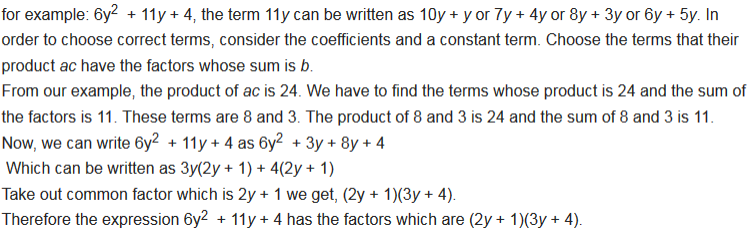Example 13
factorize 3x2 – 2x – 8 by splitting the middle term.
Solution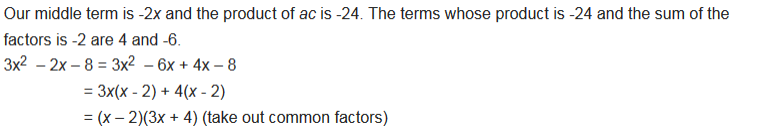Example 14
factorize x2 + 10x + 25 by splitting the middle term.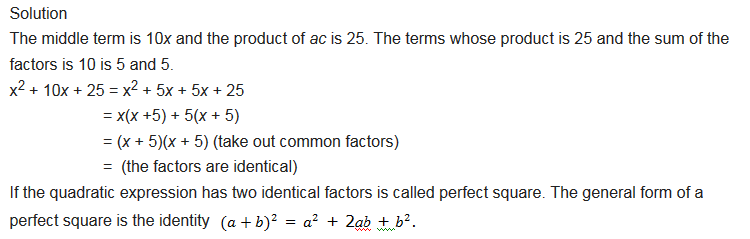### Factorization by Inspection

Example 15
factorize x2 + 3x + 2 by inspection.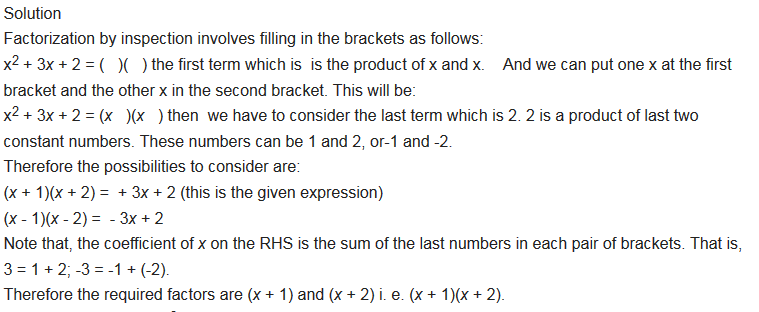Example 16
factorize 4x2 + 5x – 6 by inspection.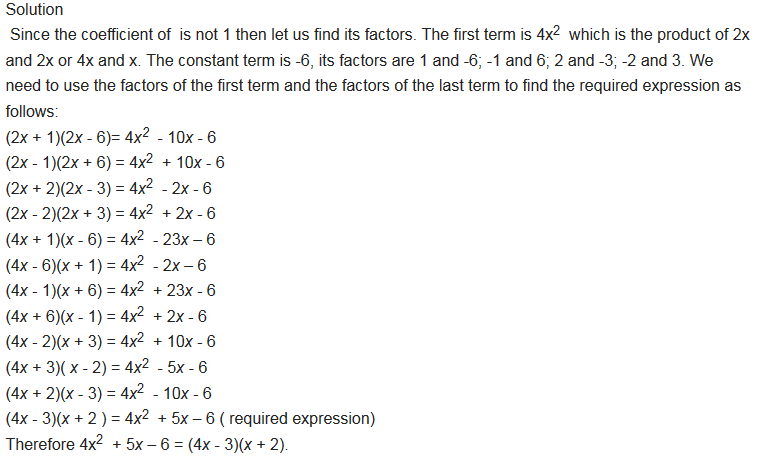Exercise 1
Factorization Exercise;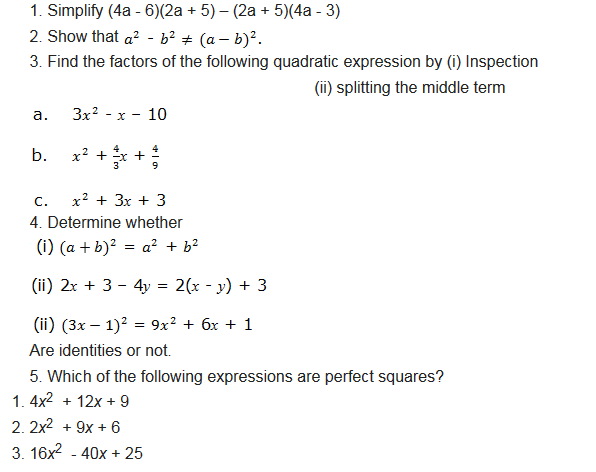### though this post please to read our notes by classes click lick button down

JE UNAMILIKI SHULE AU BIASHARA NA UNGEPENDA IWAFIKIE WALIO WENGI?BASI TUNAKUPA FURSA YA KUJITANGAZA NASI KWA BEI NAFUU KABISA BOFYA HAPA KUJUA

But for more post and free books from our site please make sure you subscribe to our site and if you need a copy of our notes as how it is in our site contact us any time we sell them in low cost in form of PDF or WORD.

### UNAWEZA JIPATIA NOTES ZETU KWA KUCHANGIA KIASI KIDOGO KABISA:PIGA SIMU:0787237719

SHARE THIS POST WITH FRIEND